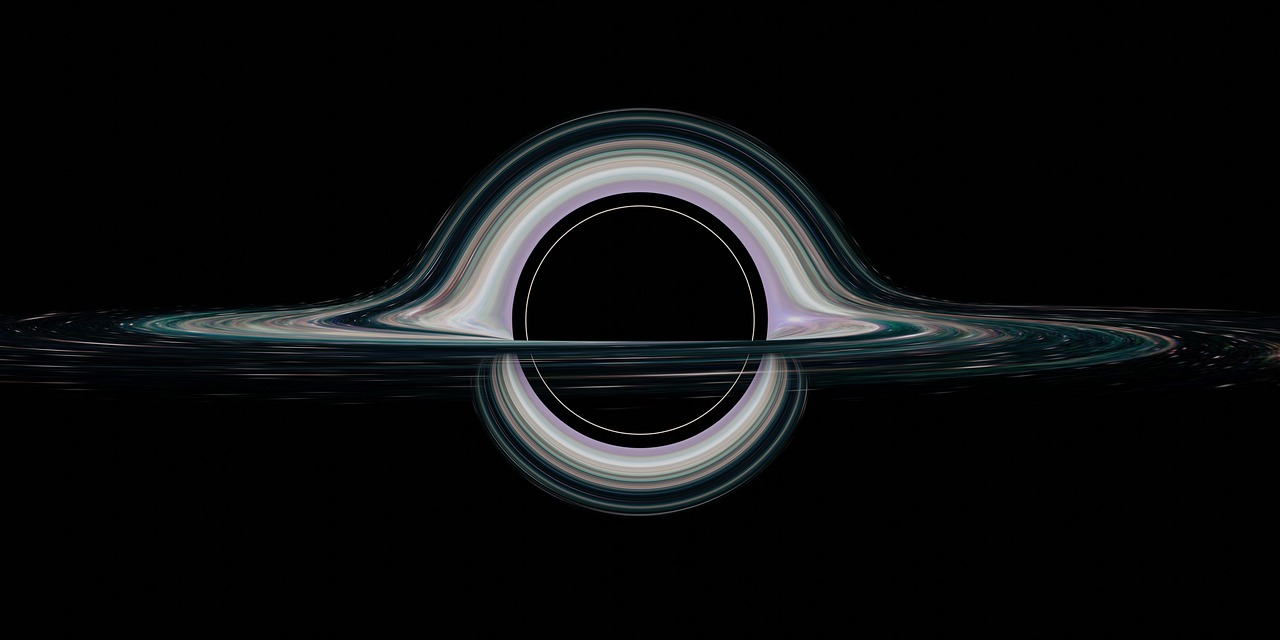# Planck Units and Black Holes

What does spacetime look like at the quantum scale? What exactly do we expect to find, perhaps the ‘atoms’ of spacetime? Does nature dare to reveal the answer to one of her most ancient enigmas: the quantum description of spacetime? Black hole physics and quantum mechanics may give us a hint to the answers to these questions.Figure 1. Pixels in spacetime. Is spacetime fundamentally pixelated like an LCD screen? Image: Gürcan Gür.

## Heisenberg vs Schwarzschild

First, let us state two known concepts:

On the one hand, the Heisenberg uncertainty principle tells us that there is a fundamental blurriness to our ability to measure multiple properties of a given system at the same time. This blurriness is constrained by the Planck constant $$\hbar$$, a very small number in our everyday units, and results, for instance, in a relation between the uncertainties in momentum (i.e. mass times velocity), $$\Delta p$$, and position $$\Delta x$$ of a particle:

$$\Delta x \Delta p \geq \frac{\hbar}{2}$$.

On the other hand, the Schwarzschild radius tells you that any massive object, like the Earth or the Sun, can become a black hole if it is squashed down to its Schwarzschild radius, which is given by the following relationship

$$r_s=\frac{2GM}{c^2}$$.

Here $$G$$ is Newton’s constant, $$M$$ the mass of the object and $$c$$ is the speed of light. Recall that we are not allowed to look beyond the Schwarzschild radius from the outside as no light can escape.

Now that we have briefly recapped these concepts, let us turn to our original quest of exploring the realm of quantum spacetime.Figure 2. A black hole. What do black holes tell us about the nature of our universe? Image: Alexander Antropov.

## Universal Units

If we wanted to explore this quantum realm we would need a highly energetic particle to probe quantum length scales of the order $$L = \Delta x$$. This particle would move very fast and hence have an energy which according to the laws of relativity would be of order  $$E = \Delta p c$$. Now if we insert these two equations into the Heisenberg relation we find

$$L \frac{E}{c} \geq \frac{\hbar}{2}$$.

Einstein taught us that energy and mass are two sides of the same coin and are simply related by his famous formula $$E=Mc^2$$. After inserting Einstein’s formula in the expression above and some rearranging one finds the following:

$$2M \geq \frac{\hbar}{Lc}$$.

That is, the energy of a quantum particle that can probe sizes of length $$L$$ needs at least a mass $$M$$ described by this equation. In addition to this Heisenberg limit of blurriness, we also need to keep in mind that if we squash something into a size $$L$$ smaller than its Schwarzschild radius $$r_s$$ we will create a black hole with an event horizon that blocks our view from the inside. This makes it impossible to say anything at even smaller scales. Therefore this leads us to another restriction, namely that

$$L > r_s = \frac{2GM}{c^2}$$.

Combining our two last equations results in

$$L > \frac{G\hbar}{Lc^3}$$.

Both sides of the equation depend on the length and hence we can solve for $$L$$ leading to

$$L> \sqrt{ \frac{G\hbar}{c^3} }= \ell_p$$,

where $$\ell_p$$ is a fixed length, obtained as a combination of constants of nature alone, known as the Planck length, which has a numerical value of

$$\ell_p \approx 1.6 \times 10^{-35}m$$.

What we have found is outstanding! By using Heisenberg’s uncertainty principle and the fact that the Schwarzschild radius shadows what happens behind the black hole horizon, we found that we are fundamentally unable to ‘see’ what happens at length scales smaller than the Planck length – meaning that the Planck length may hint at a fundamental unit of space!

Of course, this is just a small piece of a greater puzzle and does not really tell us what exactly the ‘atoms’ of spacetime are nor how they should behave. The true, yet uncharted description of quantum spacetime is still mysterious and seemingly unfathomable. But nature is inviting us to understand our quantum universe!